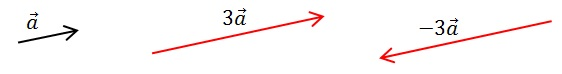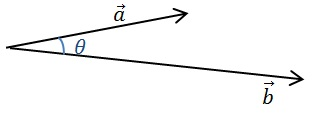# How to Multiply Vectors

We will look at three ways to multiply the vectors. First, we will look at the scalar multiplication of vectors. Then, we will look at multiplying two vectors. We will learn two different ways to multiply vectors, using the scalar product and the cross product.

## How to Multiply Vectors by a Scalar

When you multiply a vector by a scalar, each component of the vector gets multiplied by the scalar.

Suppose we have a vector$\vec{a}$, that is to be multiplied by the scalar$k$. Then, the product between the vector and the scalar is written as$k\vec{a}$. If$a>0$, then the multiplication would increase the length of$\vec{a}$ by a factor$k$.  If$a<0$, then, in addition to increasing the magnitude of$\vec{a}$ by a factor$k$, the direction of the vector would also be reversed.With regards to vector components, each component gets multiplied by the scalar. For instance, if a vector$\vec{q} = (q_x, q_y, q_z)$, then$k\vec{q} = k(q_x, q_y, q_z) = (kq_x,kq_y,kq_z)$.

Example

The momentum vector$\vec{p}$ of an object is given by$\vec{p}=m\vec{v}$, where$m$ is the mass of the object and$\vec{v}$ is the velocity vector. For an object with a mass of 2 kg having a velocity of$\left(3.8,5.4,2.6\right)$ m s-1, find the momentum vector.

The momentum is$\vec{p}=m\vec{v}=2\left(3.8,5.4,2.6\right)=\left(7.6,10.8,5.2\right)$ kg m s-1.

## How to Find the Scalar Product of Two Vectors

The scalar product (also known as the dot product) between two vectors$\vec{a}$ and$\vec{b}$ is written as$\vec{a}\cdot\vec{b}$. This is defined as,$\vec{a}\cdot\vec{b}=\left|\vec{a}\right|\left|\vec{b}\right|\cos\theta$

where$\theta$ is the angle between the two vectors if they are placed tail-to-tail as shown below:The scalar product between two vectors yields a scalar quantity. Geometrically, this quantity is equal to the product of the magnitude of one vector’s projection on the other and the magnitude of the “other” vector:Using the components of vectors along the Cartesian plane, we could obtain the scalar product as follows. If the vector$\vec{a}=\left(a_{x},a_{y},a_{z}\right)$ and$\vec{b}=\left(b_{x},b_{y},b_{z}\right)$, then the scalar product$\vec{a}\cdot\vec{b}=a_{x}b_{x}+a_{y}b_{y}+a_{z}b_{z}$

Example

Vector$\vec{p}=\left(-2,3,7\right)$ and$\vec{q}=\left(10,0,5\right)$. Find$\vec{p}\cdot\vec{q}$.$\vec{p}\cdot\vec{q}=(-20)+0+35=15$

Example

The work done$W$ by a force$\vec{F}$, when it causes a displacement$\vec{d}$ for an object is given by,$W=\vec{F}\cdot\vec{d}$. Suppose a force of$\vec{F}=\left(53,-16,0\right)$ N causes a body to move, whose displacement under the force is is$\vec{d}=\left(20,-5,0\right)$ m. Find the work done by the force.$W=\vec{F}\cdot\vec{d}=(53\times 5)+((-16)\times(-5)))+0=1140$ J.

Example

Find the angle between the two vectors$\vec{a}=\left(5,8,-3\right)$ and$\vec{b}=\left(2,0,-5\right)$.

From the definition of the scalar product,$\cos\theta=\frac{\vec{a}\cdot\vec{b}}{\left|\vec{a}\right|\left|\vec{b}\right|}$.  Here, we have$\left|\vec{a}\right|=\sqrt{5^{2}+8^{2}+(-3)^{2}}=\sqrt{98}=9.9$ and$\left|\vec{b}\right|=\sqrt{2^{2}+0^{2}+(-5)^{2}}=\sqrt{29}=5.4$

Then,$\cos\theta=\frac{\vec{a}\cdot\vec{b}}{\left|\vec{a}\right|\left|\vec{b}\right|}=\frac{(5\times2)+(8\times0)+((-5)\times(-3))}{9.9\times5.4}=\frac{10+15}{53.5}=0.28.$$\therefore\theta=\cos^{-1}(0.28)=74^{o}$.

If two vectors are perpendicular to each other, then the angle$\theta$ between them is 90o. In this case,$\cos\theta=0$ and so the scalar product becomes 0. In particular, for unit vectors in the Cartesian coordinate system, we note that,$\hat{i}\cdot\hat{j}=\hat{j}\cdot\hat{k}=\hat{k}\cdot\hat{i}=0$

For parallel vectors, the angle$\theta$ between them is 0o. In this case,$\cos\theta=1$ and the scalar product simply becomes the products of the magnitudes of the vectors. In particular,$\hat{i}\cdot\hat{i}=\hat{j}\cdot\hat{j}=\hat{k}\cdot\hat{k}=1$

The scalar product is commutative. i.e.$\vec{a}\cdot\vec{b}=\vec{b}\cdot\vec{a}$.

The scalar product is also distributive. i.e.$\vec{a}\cdot\left(\vec{b}+\vec{c}\right)=\vec{a}\cdot\vec{b}+\vec{a}\cdot\vec{c}$

## How to Find the Cross Product of Two Vectors

The cross product (also known as the vector product) between two vectors$\vec{a}$ and$\vec{b}$ is written as$\vec{a}\times\vec{b}$. This is defined as,$\vec{a}\times\vec{b}=\left|\vec{a}\right|\left|\vec{b}\right|\sin\theta$

The vector product or the cross product, unlike the scalar product, gives a vector as the answer. The above formula gives the magnitude of the vector. To get the direction of this vector, imagine turning a screwdriver from the direction of the first vector towards the direction of the second vector. The direction that the screwdriver “goes in” is the direction of the vector product.For instance, in the above diagram, the vector product is$\vec{a}\times\vec{b}$ will point into the page, whereas$\vec{b}\times\vec{a}$ will point out of the page.

Clearly, then, vector product is not commutative. Rather,$\vec{a}\times\vec{b}=-\vec{b}\times\vec{a}$.

The vector product between two parallel vectors is 0. This is because the angle$\theta$ between them is 00, making the$\sin\theta=0$.

With regards to unit vectors, we then have$\hat{i}\times\hat{i}=\hat{j}\times\hat{j}=\hat{k}\times\hat{k}=0$

Also, we have$\hat{i}\times\hat{j}=-\hat{j}\times\hat{i}=\hat{k}$$\hat{j}\times\hat{k}=-\hat{k}\times\hat{j}=\hat{i}$$\hat{k}\times\hat{i}=-\hat{i}\times\hat{k}=\hat{j}$

With regards to components, the vector product is given by,

$latex \vec{a}\times\vec{b}=\left|\begin{array}{ccc} \hat{i} & \hat{j} & \hat{k}\\ a_{x} & a_{y} & a_{z}\\ b_{x} & b_{y} & b_{z} \end{array}\right|&s=1$$=\left(a_{y}b_{z}-a_{z}b_{y}\right)\hat{i}+\left(a_{z}b_{x}-a_{x}b_{z}\right)\hat{j}+\left(a_{x}b_{y}-a_{y}b_{x}\right)\hat{k}$

Example

Find the cross product between vectors$\vec{a}=\left(3,-1,0\right)$ and$\vec{b}=\left(-2,4,2\right)$.$\vec{a}\times\vec{b}=\left((-1\times2)-(0\times4)\right)\hat{i}+\left((0\times-2)-(3\times2)\right)\hat{j}+\left((3\times4)-(-1\times-2)\right)\hat{k}=\left(-2,-6,10\right)$.